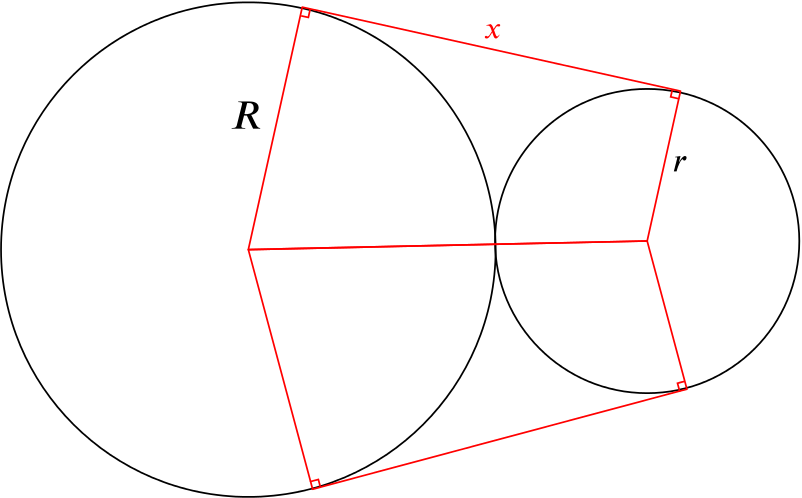Food for thought

## Suggestion

A piece of thin wire of length $L$ binds together two cylindrical welding rods, of radii $R$ and $r$, by passing all the way around them both.

Find $L$ in terms of $R$ and $r$.

What are the types of shape that the wire takes?

Look atTwo cylindrical welding rods with part of the wire drawn in

Can you see any way of drawing in an extra line to make a right-angled triangle with two side lengths you know? Does the angle of this triangle help you to work out what the lengths of the other parts will be?